Awschalom Group
Gigahertz Dynamics of a Strongly Driven Single Quantum Spin

Two-level quantum systems in a solid-state environment are an attractive platform to perform fundamental studies of a single quantum object as well as to develop emerging quantum information technology. An important figure of merit is the ratio of the time it takes to manipulate the system compared with its coherence time. Sufficiently large ratios may enable quantum error correction, motivating efforts to increase the coherence time and decrease the manipulation time. Here we focus on reducing the manipulation time of nitrogen vacancy center spins in diamond to gigahertz rates.

The nitrogen vacancy (NV) center is a spin-1 diamond lattice defect with energy levels shown below.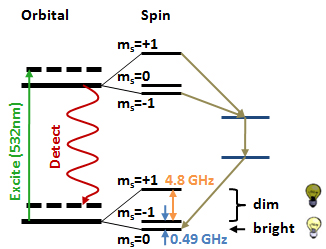For our time-domain measurements, we initialize the NV center optically, manipulate the spin using a microwave frequency driven field in the dark, and then read out the spin state using spin-dependent photoluminescence. The measurement duty cycle is shown below and to the left. The microwave driving fields are applied using a short-terminated coplanar waveguide (shown below and to the right).The microwave pulse will cause the spin to evolve from the initialized state (P = 1) according to Rabi’s formula: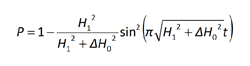where H1 is the amplitude of the rotating field, ΔH0 is the detuning from the transition frequency, t is time, and P is the spin population. P = 1 corresponds to the ms = 0 state and P = 0 corresponds to the ms = -1 state.

Conventional understanding of driven two-level dynamics is described in terms of a pseudospin ½ in a Larmor field, H0. The spin is driven by an oscillating field that is the sum of two fields rotating in opposite directions. One field rotates with the spin, applying a constant torque, and produces a net rotation which induces Rabi oscillations between the two energy eigenstates. As long as the counter-rotating field is small compared to H0, it has a negligible effect since it exerts a rapidly varying torque that averages itself out over time. Neglecting the counter-rotating term is the basis of the rotating wave approximation.

In the standard spin resonance regime, we expect sinusoidal oscillations between photoluminescence intensity (IPL) of the ms = 0 (P = 1) and ms = -1 (P = 0) state at a frequency given by H1IPL for each state was calibrated using an adiabatic passage technique. Below is a plot of the Rabi frequency measured as a function of the microwave power, with the solid line showing a square root trend. The Rabi field for the red open circles are calculated from the microwave power because the data are not sinusoidal for these values of driving power.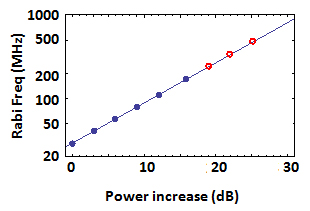The experimental Rabi oscillations are smooth and sinusoidal for small driving fields (H1 = 29 MHz below). As we drive the spin harder, anharmonic components emerge causing the P to stall for a few pulse widths (H1 = 223 MHz below). For strongly driven spins, the result is anharmonic and the spin rotates much faster than expected from just the co-rotating component of the driving field (H1 = 440 MHz below).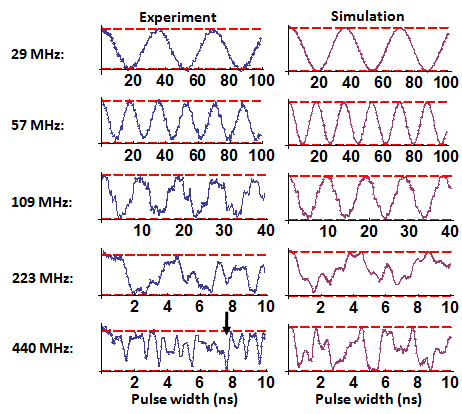In such a regime, the co- and counter-rotating fields can act either in concert or in opposition resulting in a rapid change or a stalling of P. This can result in a complete spin flip in less than 0.5 ns, as shown by the arrow above for H1 = 440MHz.

Simulations were also performed separately using each experimentally measured microwave pulse to, shown above and to the right. These account for transient effects in the experiment, and show excellent qualitative agreement with the experimental data.

The strongly driven pulses are short and intense, resulting in the leading and trailing edges having substantially more influence than a standard spin resonance experiment. To mitigate the effect of these pulse edges, we drive the spin using Gaussian-shaped pulses, normalized to have the same full-width at half maximum of the square pulses. The result of these pulses are shown below, along with the simulations of the final population. At H1 = 109 Hz the Gaussian pulses recover sinusoidal Rabi oscillations, in contrast to the step-like oscillations seen for the square pulses. As H1 increases, two separate regimes begin to emerge: one where flips occur within the subperiod duration (W < 2 ns) and another at longer pulses. The short pulse duration regime is generally anharmonic whereas the longer pulses show comparitively smooth Rabi oscillations.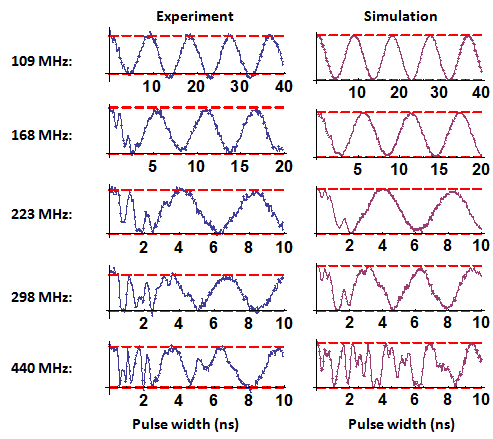An important feature from these data are the reproducible sub-nanosecond spin flips. The simulations demonstrate that while the microwave pulses have non-resonant tails longer than a nanosecond, sub-nanosecond spin flips are still achievable. By combining these sub-nanosecond manipulation rates with the millisecond coherence time in diamond, more than a million coherent operations can be performed on a single NV center spin at room temperature.

To learn more about our studies, please refer to "Gigahertz Dynamics of a Strongly Driven Single Quantum Spin", G. D. Fuchs, V. V. Dobrovitski, D. M. Toyli, F. J. Heremans, and D. D. Awschalom, Science 326, 1520 (2009).